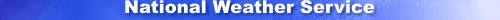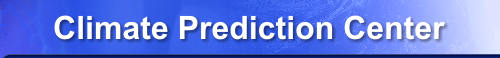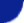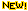Site Map News Organization SearchHOME > Monitoring_and_Data > Oceanic and Atmospheric Data > Reanalysis: Atmospheric Data > wgrib2-time_processing

wgrib2: -time_processingThe -time_processing option is being introduced with wgrib2 v2.0.7 and has a superset of the capablities of -ave and -fcst_ave. The new -ave and -fcst_ave options just call the -time_processing option.

Introduction

Product Definintion Templates (PDT) come in 3 variaties. Type A is an uncommon PDT and only has a reference time with no forecast information. Typically they are for observations such as radar or satellite data. Type B has a reference time with a forecast time. For example, the reference time could be Januay 1, 2000 at 00Z. The forecast time could be a 120 hour forecast. Typically the reference time is initial time of the forecast but Table 1.2 allows you to specify the significance of the reference time. Type B specifies a point in time. Finally Type C is similar to Type B except it is for a continous or non-continuous time interval. Some examples of type C PDTs,
• average temperature of a 0-6 hour forecast
• maximum temperature of 00Z-24Z observations taken every hour
• maximum temperature of 00Z-24Z observations (as from a min-max thermometer)
• average 00Z temperature for the month of May (analysis)
• standard deviation of 00Z temperature of month of May 120 hour forecast
• 30 year climatalogy of average June temperature analyses

The interesting part of Type C PDTs is that the format is in terms of a stackable temporal operator. For example, a simple height forecast (ex 12 hour forecast) can be thought of as a height(time1, time2) where time1 is the intitial time of the forecast and time2 is the forecast time. The possible temporal averaging operators can be

ave1 = 1/N(H(t0,t1) + H(t0+dt,t1) + ... + H(t0+(N-1)*dt,(N-1)))
ave2 = 1/N(H(t0,t1) + H(t0,t1+dt) + ... + H(t0,(N-1)*dt))
ave3 = 1/N(H(t0,t1) + H(t0+dt,t1-dt) + ... + H(t0+(N-1)*dt, t1-(N-1)*dt))
ave4 = 1/N(H(t0,t1) + H(t0-dt,t1+dt) + ... + H(t0-(N-1)*dt, t1+(N-1)*dt))

the continous case is denoted by having dt == 0
By stacking the averaging operator, a monthly climatology can be described by a 30 year average of a monthly average.

The Type C PDTs allow 11 standard temporal operations (code table 4.10) which include the commonly used average, accumulation, maximum, minimum, RMS and standard deviation.

The -time_processing option will allow you to calculate the average/minimum/maximum/standard_deviation of a time series of Type B or Type C PDTs. Only a limited number of operators are supported. The total set is given by Code Table 4.10 Only a limited number of types of time intervals as defined by Code Table 4.11 are supported.

Usage

-time_processing Code_Table_4.10 Code_Table_4.11 (time interval)  (output grib file)
Code_Table_4.10:  0 or ave, computes average
2 or max, computes maximum
3 or min, computes minimum
5 or rms, computes the root mean square
6 or std_dev, computes sample standard deviation
Code_Table_4.11:  1 or analyses,  time series of set of analyses/forecasts
2 or forecast,  time series from one (long) forecast
(time interval):  (integer)(units)
units:            hr, dy, mo, yr, mn (minutes, v2.0.8)

Code Table 4.11 = 1 (analyses)

When Code Table 4.1 is set to 1, the input fields have to be processed in a special order. Suppose that you want to make a daily average from 4 analyses spaced at 6 hours. Then you process 4 fields with reference times incrementing by 6 hours with the same forecast time, variable, level and grid. Whenever the field is unexpected, a new average is made. Note: the -time_processing option will handle missing fields. For example,
Code Table 4.10 = 0, Code Table 4.11 = 1

U500 2000-01-02 00Z             start ave
U500 2000-01-02 06Z
U500 2000-01-02 12Z
U500 2000-01-02 18Z             end ave
V500 2000-01-02 00Z             start ave
V500 2000-01-02 06Z
V500 2000-01-02 12Z
V500 2000-01-02 18Z             end ave
Z500 2000-01-02 00Z             start ave
Z500 2000-01-02 06Z
Z500 2000-01-02 12Z
Z500 2000-01-02 18Z             end ave
Code Table 4.1 = 1 is good for making means of many analyses.

Code Table 4.11 = 2 (forecast)

When Code Table 4.1 is set to 1, the input fields have to be processed in a special order. Suppose that you want to make a daily average from one forecast with using forecast hour = 0, 6, 12 and 18. (The forecast for the first day.)

Code Table 4.10 = 0, Code Table 4.11 = 2

U500 start 2000-01-02 00Z fhour=00 hours    start ave
U500 start 2000-01-02 00Z fhour=06 hours
U500 start 2000-01-02 00Z fhour=12 hours
U500 start 2000-01-02 00Z fhour=18 hours    end ave
V500 start 2000-01-02 00Z fhour=00 hours    start ave
V500 start 2000-01-02 00Z fhour=06 hours
V500 start 2000-01-02 00Z fhour=12 hours
V500 start 2000-01-02 00Z fhour=18 hours    end ave
T500 start 2000-01-02 00Z fhour=00 hours    start ave
T500 start 2000-01-02 00Z fhour=06 hours
T500 start 2000-01-02 00Z fhour=12 hours
T500 start 2000-01-02 00Z fhour=18 hours    end ave
Code Table 4.11 = 2 is good for processing a single forecast run.

Code Table 4.11 = 3, 4, 5

These values of Code Table 4.11 are not commonly used and are not supported.

Code Table 4.10 = 0 (average)

Code Table 4.11 = 0 means the -time_processing option will compute the mean. This is the most common usage. If you are trying to compute the average and some fields are missing, then average will be computed from the available fields and the missing field value will reflect the number of missing fields. If some grid values are undefined, then the average for the grid value will be undefined as there is no mechanism to so show that the average was computed with fewer fields.

Code Table 4.10 = 2 (maximum)

Code Table 4.11 = 2 means the -time_processing option will find the maximum value. If you are trying to find the maximum and some fields are missing, then the maximum will be found available fields and the missing field value will reflect the number of missing fields. If some grid values are undefined, then the maximum for the grid value will be undefined as there is no mechanism to so show that the maximum was derived from fewer fields.

Code Table 4.10 = 3 (minimum)

Code Table 4.11 = 3 means the -time_processing option will find the minimum value. If you are trying to find the minimum and some fields are missing, then the minimum will be found available fields and the missing field value will reflect the number of missing fields. If some grid values are undefined, then the minimum for the grid value will be undefined as there is no mechanism to so show that the minimum was derived from fewer fields.

Code Table 4.10 = 3 (root mean square)

Code Table 4.11 = 3 means the -time_processing option will compute the root mean square (rms). The mean of the square of the grid values is computed. Then the square root is saved in the grib message. The value is marked as undefined if any of the individual values is undefined.

Code Table 4.10 = 6 (standard deviation)

Code Table 4.11 = 6 means the -time_processing option will find the sample standard devation. Welford's method for computing the mean and variance is used because it is a one-pass scheme with the accuracy of a two-pass algorithm.

Code Table 4.10 = 1, 4, 7 8, 9, ..

These are not supported. Although adding support for 1, 4, 7, 8 and 11 seems easy. However, I have not seen these values used.

-ave (dt) (output)

This option has been replaced by a macro that calls "-time_processing 0 1 (dt) (output)".

-fcst_ave (dt) (output)

This option has been replaced by a macro that calls "-time_processing 0 2 (dt) (output)".

Minutes and Seconds

There is no minutes time unit. Wgrib2 uses the standard GrADS names for time units which means the "mn" is the unit for minutes. Unfortunately it is too easy to confuse "mn" with the month time unit. When there is a real need for minutes, then "mn" will be added.

Fast Averaging

Suppose we have a month of analyses at 3 hour intervals and want to make a monthly mean for Nov. 2014. Using the above approach, the steps would be

1.  cat narr.201411????.grb2 >tmp.grb2
2.  wgrib2 tmp.grb2 |  \
3.     sort -t: -k4,4 -k5,5 -k6,6 -k3,3 | \
4.     wgrib2 tmp.grb -i -set_grib_type c3 -ave 3hr narr.201411

The first line creates a file with all the data.
The second line make an inventory.
The third line sorts the inventory in the order for -ave to process.
The fourth line makes the average by processing data in the order
determined by the inventory created by line 3.

The above approach processes one average at a time and requires a minimal amout of memory. However, if you count the I/O operations, you find that there are 4 I/O operations for every field as well as the writes of the monthly means. In addition, the read (line 4) is random access.

HPC file systems are very fast for large files that are read sequentially. On the other hand, HPC file systems are horrible for small random access reads like in the previous example. Making monthly means by averaging 3 hourly NARR data was taking about three quarters of an hour on a multi-million dollar machine. The problem was that the file system was optimized for large sequential reads rather than small random-access reads. The following shows another approach.

1.  cat narr.201411????.grb2 | \
2.     wgrib2 - \
3.        -if_fs ":HGT:200 mb:" -ave 3hr narr.201411 \
4.        -if_fs ":UGRD:200 mb:" -ave 3hr narr.201411 \
5.        -if_fs ":VGRD:200 mb:" -ave 3hr narr.201411 \
6.        -if_fs ":TMP:200 mb:" -ave 3hr narr.201411

The first line copies the data in chronological order and
writes it to the pipe.
The second line has wgrib2 read the grib data from the pipe.
The third line selects the Z200 fields and runs the averaging
option on it.  We are assuming the narr.* fields only have
one Z200 field and narr.201411???? puts the data into
chronological order.
Lines 4-6 apply the averaging option to other fields.

The above approach computes the mean of Z200, U200, V200 and T200 data at the same time with the use of more memory. The I/O consists of sequential read of all the files and the writes of the monthly means. The above script only creates the mean of Z200, U200, V200 and T200 but you could write a very long command line and compute the mean of all the fields in the file. Here are the guts of a bash script, fast_grib2_mean.sh, which creates and runs the command line.

1.  wgrib2 \$1 -match_inv | cut -f4- -d: | sed -e 's/:n=.*//' >\$tmp
2.  cmd="cat \$* | \$wgrib2 - -set_grib_type c3 "
4.  do
5.    cmd="\$cmd -if_fs '\$line' -ave \$dt \$out "
6.  done <\$tmp
7.  eval \$cmd

1. \$1 is the first file to average.
Line 1 creates a file with the field names (minus date codes)
2. cmd is the command line that is being built
3. loop over all the lines in file \$tmp
5. generate the "-if_fs/-ave" for the cmd line
Older versions of the web paged used -if but that caused problems when
\$line included metacharacters such as parentheses.
6. bash syntax to have the while loop read from \$tmp
7. run the command line

Making the NARR monthly means using the above approach uses large sequential reads which is optimal for the HPC file system. The run time went from 3/4 of an hour to maybe a minute.

Fast Forecast Averaging

The previous shell script was for a fast averaging of many analyses. Sometimes one want to average several forecasts starting from the same initial time. An example would producing a week-4 forecast.

1.  \$wgrib2 \$1 -match_inv | cut -f4-5 -d:  >\$tmp
2.  cmd="cat \$* | \$wgrib2 - -set_grib_type c3 "
4.  do
5.    cmd="\$cmd -if_fs '\$line' -fcst_ave \$dt \$out "
6.  done <\$tmp
7.  eval \$cmd

1. \$1 is the first file to average.
Line 1 creates a file with the name and level for each field
It is assumed that the name and level is unique in the file.
2. cmd is the command line that is being built
3. loop over all the lines in file \$tmp
5. generate the "-if_fs/-fcst_ave" for the cmd line
Older versions of the web paged used -if but that caused problems when
\$line included metacharacters such as parentheses.
6. bash syntax to have the while loop read from \$tmp
7. run the command line

Using the -merge_fcst option in a like manner to the -fcst_ave option. in a like

Monthly Climatologies

Once you can make an average, making a monthly climatology should be easy. Except it isn't. Here are some of the problems that I encountered.
1. February has 28 days except when it doesn't. This causes problems because wgrib2 -ave will not average 28 and 29 day intervals.
2. '116@6 hour ave(anl)' includes a regex metacharacter
3. the process id changed
4. the subcenter changed
The solutions were:
1. rewrite the grib file with
-if_fs '116@6 hour ave(anl)' -set_ftime2 '112@6 hour ave(anl)' -fi \
-if_fs '116@6 hour ave(6 hour fcst)' -set_ftime2 '112@6 hour ave(6 hour fcst)' -fi \
-if_fs '116@6 hour ave(3-6 hour acc fcst)' -set_ftime2 '112@6 hour ave(3-6 hour acc fcst)' -fi \
2. Use -if_fs instead of -if
3. rewrite the file with -set analysis_or_forecast_process_id 180
4. rewrite the file with -set subcenter 0

Finding items 3 and 4 was a pain. Using undocumented option v98 helps. However, the mismatches can now be uncoverted by using a verbose mode (>0) and wgrib v2.0.6.

Limitations

Fast averaging has limits impossed by wgrib2. For example, there is a limit in the maximum number of -if/-if_fs clauses. Wgrib2 v2.0.6 can process up to 2000 -if and 2000 -if_fs options. Wgrib2 v2.0.6 can accept 10000 words on the command line. Since each -if_fs/-ave clause takes 5 words on the command line and you need to include the name of the input file, you get a limit of 999 -if_fs/-ave clauses. To speed up the code, the evaluation of the -if/-if_fs options are done in parallel.

Fast averaging has limits impossed by the computer memory because Fast averaging uses multiple calls to the time_processing option. Each time_processing option requires computer memory. For example, to compute the average, you need to keep arrays for the running sum and number of times the sum was incremented. So it is possible for the fast averaging could use up too much memory.